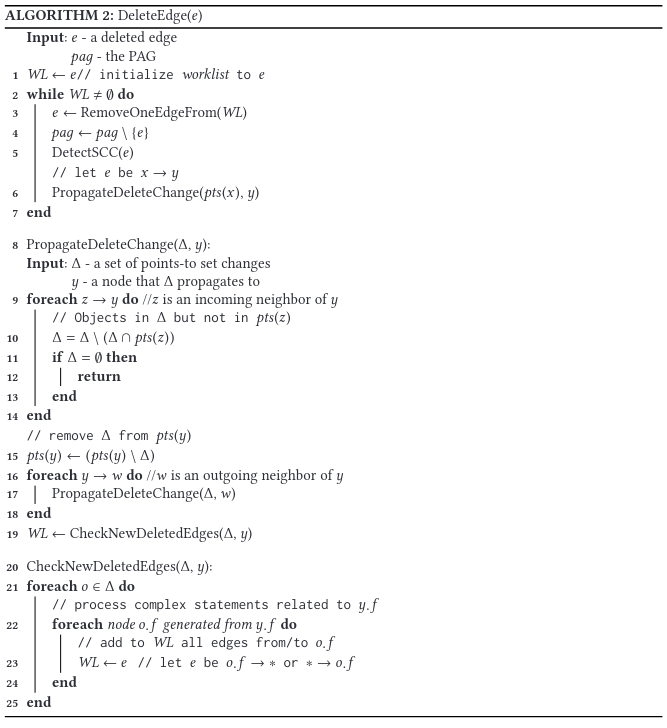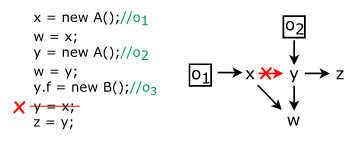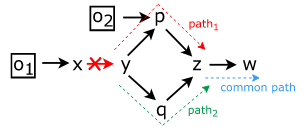# Rethinking Incremental and Parallel Pointer Analysis

Rethinking Incremental and Parallel Pointer Analysis来自TOPLAS，介绍了一种增量的可并行的指针分析算法。

### Abstract

``````1 Δ1 ← ∅;// Δ1: the new points-to constraints in each iteration
2 Δ2 ← initial method call targets;
3 while Δ2   ∅ do // repeat until Δ2 is empty
4 	Δ1 = extractNewMethodCallConstraints(Δ2)
5 	Δ2 = runAndersensAnalysis(Δ1)
6 end``````

• `reset-recompute`，例如：
• `reachability based`，例如：

### New Incremental Algorithm

Lemma 1 [Incoming Neighbors Property]. Consider an acyclic PAG and a pointer node q of which an object o ∈ pts (q). If q has an incoming neighbor r (that is, there exists an edge r →q) and o ∈ pts (r ), then there must exist a path from o to r without going through q.

Lemma 2 [Outgoing Neighbors Property]. Consider an acyclic PAG and a pointer node q of which an object o ∈ pts (q). Assume that q has an outgoing neighbor w (i.e., there exists an edge q → w) and w has an incoming neighbor r (different from q) such that o ∈ pts (r ). If r cannot reach q, then at least one of the following two conditions (or both) must hold in the PAG:

(1) There exists a path from o to w without going through q.

(2) There exists a path from q to r .• 由于`y`的其他入度不包含`o1`，所以`o1`可以从`y`的指向集中删除（Lemma 1）；
• 继续考察`y`的出度：
• 对于`z`，由于`z`的其他入度同样不包含`o1`，所以同样删除；
• 对于`w`，存在一条`o1 -> x -> w `的路径，所以这个修改会被跳过，并且终止传播（Lemma 2）；#### Parallel

Lemma 3 [Change Idempotency Property]. For an edge insertion or deletion, the update to each points-to set is an idempotent operator. In other words, if the change propagates to a node more than once from different paths, the effect of the change (i.e., the modification applied to the corresponding points-to set) must be the same.### DISCUSSIONS

• Flow sensitivity

• Context sensitivity